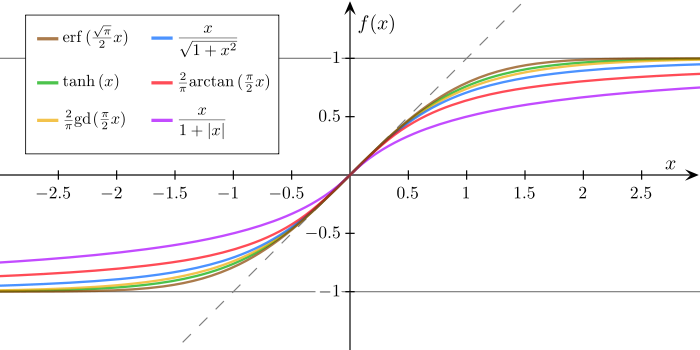Show Question
Logistic sigmoid$\sigma(x) = \frac{1}{1+e^{-x}}$

The logistic sigmoid:The logistic function is a special case sigmoid function.

A sigmoid function is a mathematical function having an "S" shaped curve (sigmoid curve). A wide variety of sigmoid functions have been used as the activation function of artifical neurons. They are used to produce the$\phi$ parameter of a Bernoulli distribution.

A collection of sigmoid functions, normalized to have a gradient of 1 at the origin: